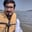Related Tags

pandas
concat
communitycreator

# How to stack two series vertically and horizontally in pandasArslan Bajwa

pandas’s library allows two series to be stacked as vertical and horizontal using a built-in command called concat(). This action is usually performed to create a dataframe from two series.

## Syntax

pandas.concat(objs, axis, join, ignore_index,
keys, levels, names, verify_integrity,
sort, copy)             

## Parameters

1. objs: This is the mapping of Dataframe or Series objects. If we pass the mapping, their keys will be sorted and used in argument keys. If keys are already passed as an argument, then those passed values will be used. Any Null objects will be dropped.

2. axis: This is the axis along which we want to stack our series. The default is 0.

0 represents Horizontal.

1 represents Vertical.

3. join: This parameter tells us how to handle indexes on other axes. It has two types: inner and outer, and the default is outer.

4. ignore_index (bool): This tells whether or not to ignore index values. The default is False.

5. keys(sequence): This tells us the sequence of the identifier in resultant indices. The default is None.

6. levels (list of sequences): This tells us the levels for constructing Multi-index. The default is None.

7. names (list): This tells us the names of levels in resultant index. The default is None.

8. verify_integrity (bool): This checks duplicates in the resultant axis. The default is False.

9. sort (bool): This sorts non-concatenation axis. It only works with outer join, and the default is False.

10. copy (bool): This tells whether or not to stop copying unnecessary data. The default is True.

## Return values

• Series: If objs contains all series.

• Dataframe: If objs contains at least one Dataframe.

## Vertical stacking

### Using concat()

#importing pandas library
import pandas as pd

#initializing two pandas series
series1 = pd.Series(range(len('educative')))
series2 = pd.Series(list('educative'))

#vertical stack with axis = 0 as parameter
print(pd.concat([series1, series2], axis = 0))


### Using append()

#importing pandas library
import pandas as pd

#initializing two pandas series
series1 = pd.Series(range(len('educative')))
series2 = pd.Series(list('educative'))

#Vertical stack using append
print(series1.append(series2))


## Horizontal stacking

#importing pandas library
import pandas as pd

#initializing two pandas series
series1 = pd.Series(range(len('educative')))
series2 = pd.Series(list('educative'))

#Horizontal stack with axis = 1 as parameter
print(pd.concat([series1, series2], axis = 1))


RELATED TAGS

pandas
concat
communitycreator

CONTRIBUTORArslan Bajwa
RELATED COURSES

View all Courses

Keep Exploring

Learn in-demand tech skills in half the time